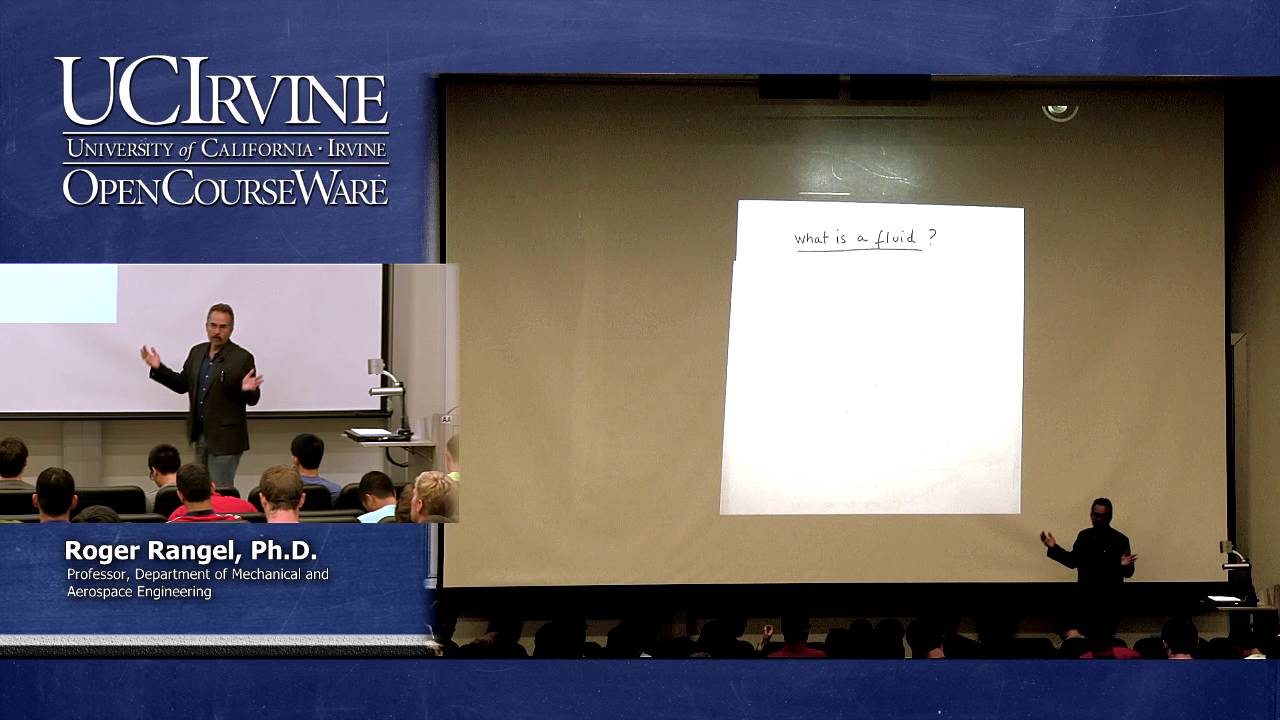UCI OpenCourseWare

# Introduction to Fluid Mechanics

This course covers fundamental concepts of fluid mechanics; fluid statics; fluid dynamics; Bernoulli's equation; control-volume analysis; basic flow equations of conservation of mass, momentum, and energy; differential analysis; potential flow; viscous incompressible flow.

Instructor

###### Professor Roger RANGEL.
Mechanical engineering
2013

#### Lecture 01 - Introduction and Fundamentals